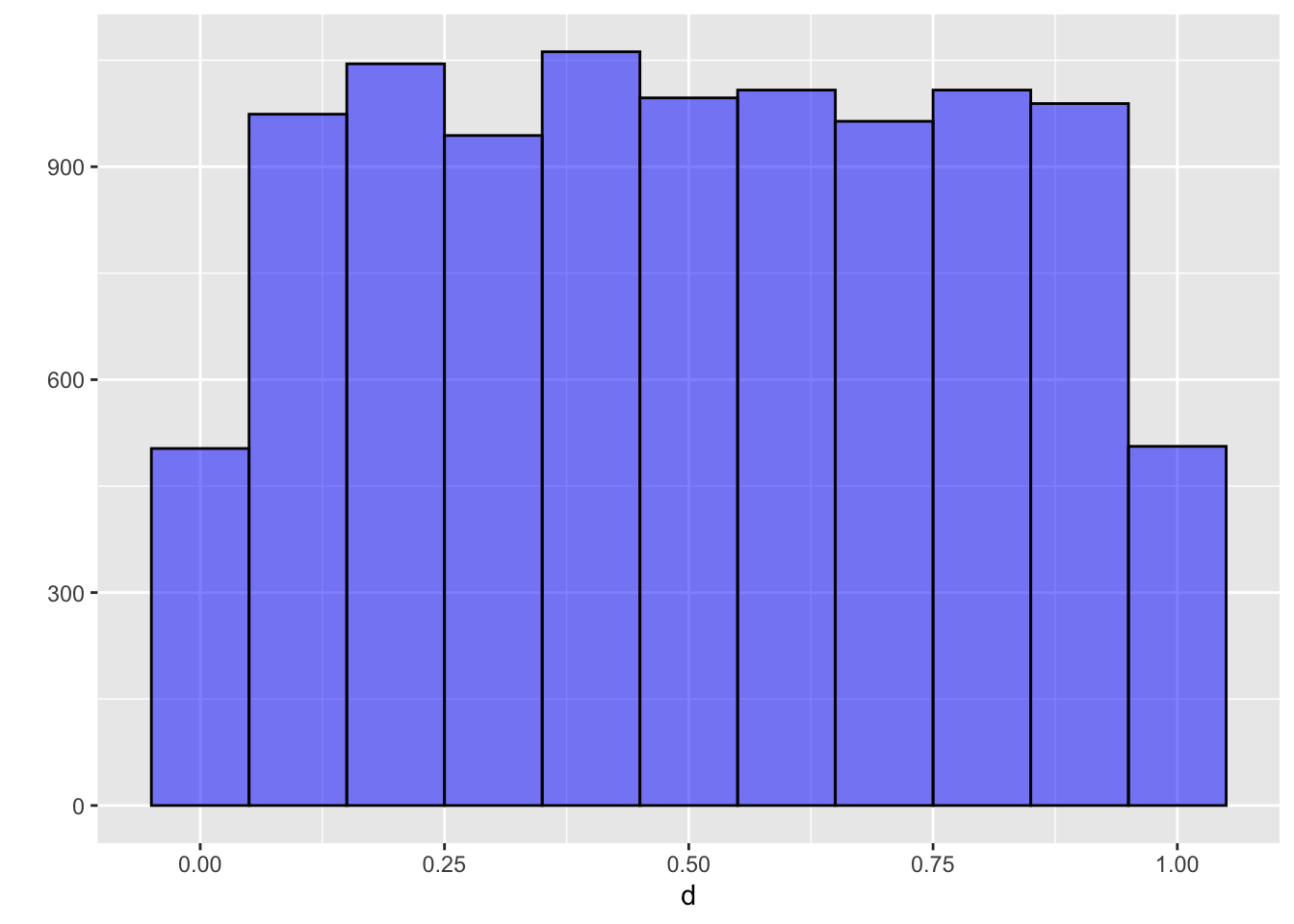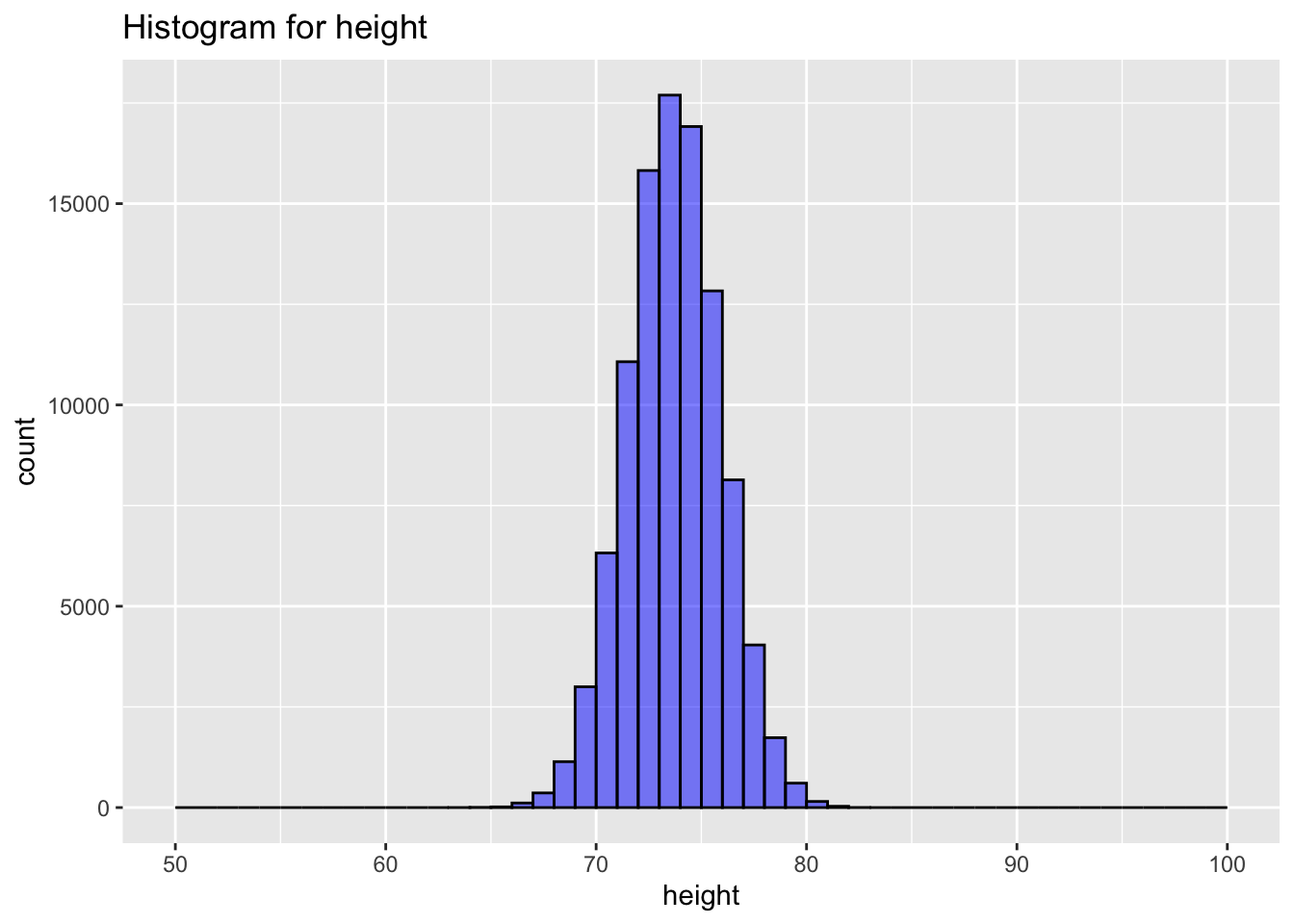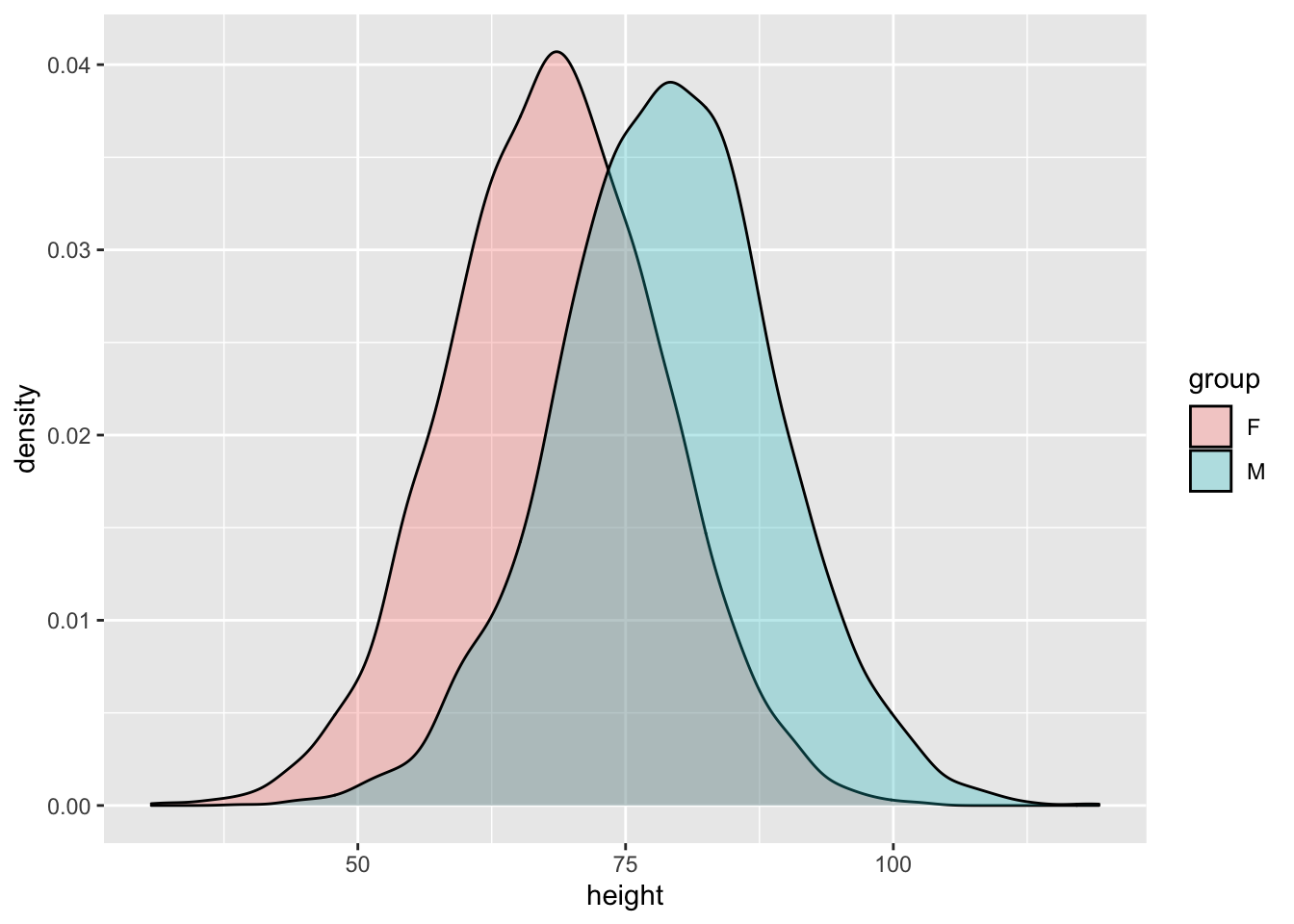# Richard Sprague

My personal website

# R-tude: Averages and Standard Deviations

Created: 2018-11-30 ; Updated: 2018-11-30

An etude is a short musical composition intended for intensive practice on a particular skill. My R-tudes are similar exercises to help me develop my R skills.

library(tidyverse)

Let’s say you have a large population of people, each of differing abilities on a scale from 0 to 1, and let’s start with the assumption that it’s completely random. Some people are higher, some lower, and the number at each level are roughly equal.

d <- runif(10000)
qplot(d,bins = 10, binwidth = 0.1, geom = "histogram", fill = I("blue"), col = I("black"), alpha = I(0.5))It’s random, so you have roughly the same number of items in each histogram bucket.

This kind of distribution makes sense in, say, a world where people are choosing a number from 1 to 100 or something completely random. But that’s not a typical real world situation. A more typical example might involve, say, height:

people_data <- data_frame(height = rnorm(100000,mean = (78.9 + 68.5) / 2, sd = (2.24 + 2.16) /2),
person = 1:100000)
## Warning: data_frame() is deprecated as of tibble 1.1.0.
## Please use tibble() instead.
## This warning is displayed once every 8 hours.
## Call lifecycle::last_warnings() to see where this warning was generated.
ggplot(data = people_data, aes(height)) +
geom_histogram(fill = "blue", col = "black", alpha = 0.5, bins = 20, breaks = seq(50,100)) +
labs(title = "Histogram for height")The spread of the curve depends on the standard deviation. The standard error of the mean for Americans is about 2.2 according to the CDC.

# Subpopulations

The above example puts everybody in the same pile. Now let’s consider what happens when the people are divided into groups. For example, let’s assume half the people are in the ‘M’ group and half in the “F” group.

pop_size = 10000

people_data <- data_frame(person = 1:pop_size,
group = factor(ifelse(rbinom(1:pop_size,1,.5),"M","F")),
height = rnorm(pop_size,mean = (78.9 + 68.5) / 2, sd = (2.24 + 2.16) /2)
)

m <- people_data[people_data$group == "M",]$height %>% length()
f <- people_data[people_data$group == "F",]$height %>% length()

people_data[people_data$group=="M","height"] <- rnorm(m,mean=78.9,sd=10) people_data[people_data$group=="F","height"] <- rnorm(f,mean=68.5,sd=10)

ggplot(data = people_data, aes(height,fill=group)) +
geom_histogram( col = "black", alpha = 0.5, bins = 20, breaks = seq(50,100)) +
labs(title = "Histogram for height")ggplot(data = people_data, aes(height,fill = group)) +
geom_density(alpha = 0.3)You can watch this on an interactive graph at: